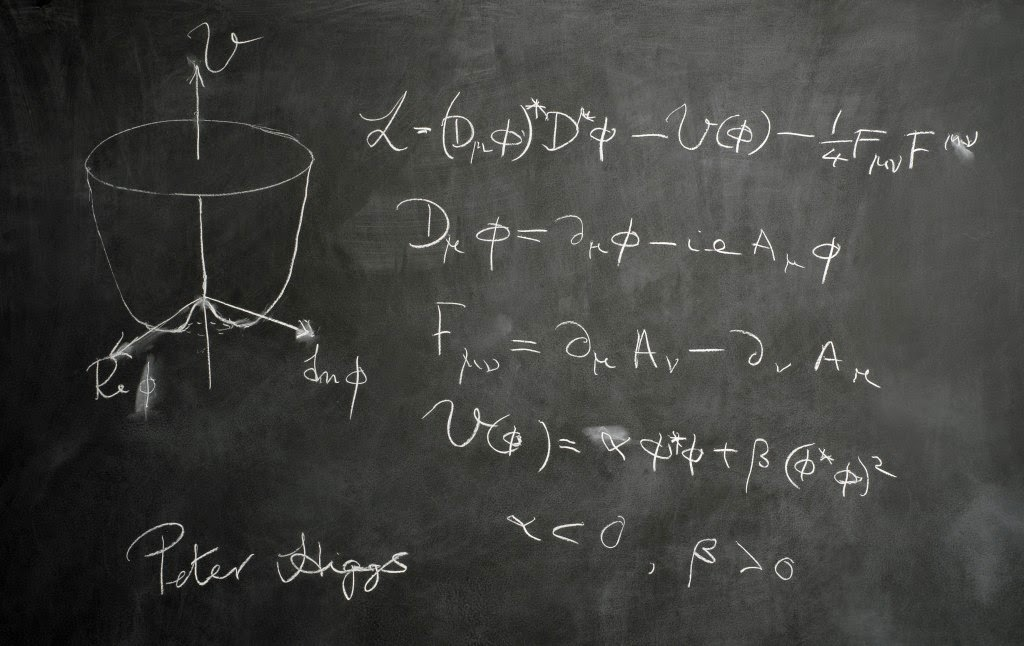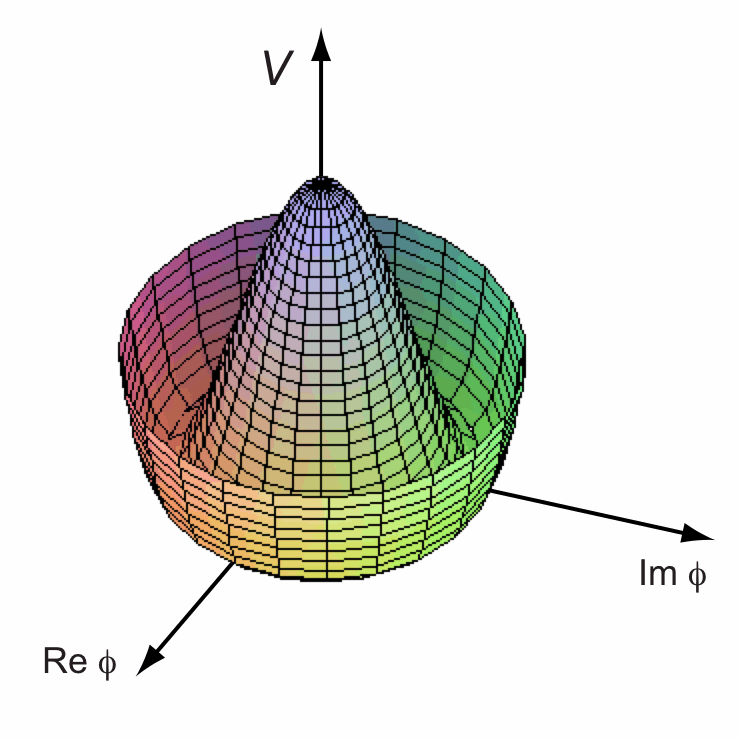### Symmetry breaking & creation of the Higgs field.

Symmetry breaking

The way in which a kind of force is acquired by the Higgs field is important and is thought to be by "symmetry breaking".

The concept is based on the theory of "phase transitions" that was described by Landau. This is analogous in some ways to the way that within a magnet, the magnetic particles (atoms) will align in an ordered (i.e. non-random, or an asymmetric, way) and jointly generate a magnetic field - but only below a certain "transition" temperature. If the magnet is heated to a temperature above this transition temperature then the particles become unaligned, arranged in a random way. There is then an overall "symmetry" of the collection of particles and in this state the overall "order parameter" is said to be zero. In a magnet, this order parameter is equivalent to the degree of "magnetisation".  When the temperature is below the transition temperature, the order parameter is regarded as non-zero (i.e. there has been an overall loss of symmetry and an overall gain in the amount of overall order in the collection of particles). This "symmetry breaking", i.e. gain in ordered alignment of the system, is believed to apply not only to magnets but also to the Higgs field.

Importantly, symmetry breaking is also the explanation of a non-zero value for the Higgs field's order (rather like magnetisation) and its ability to confer mass upon some particles. The system's energy tends to fall to the lowest possible level, which, once the universe had cooled to below the transition temperature (of e.g. a trillion degrees), results in an order parameter for the field that is not zero.  In this way, the system gains order (or field strength) and becomes "asymmetric".

The maths...

The maths that covers the "symmetry breaking" involves an equation that, prior to its use by Prof Peter Higgs, was used by Landau, although he was describing magnetisation (rather than the Higgs field). A modified version of it, replacing magnetisation (i.e. M) by the Higgs field order parameter (like field strength) (i.e. phi), was used by Higgs and can be seen in the lower half of Peter Higgs' blackboard, when he was delivering one of his scientific lectures:Image from Edinburgh University, Scotland, UKImage of the full blackboard, from Prof Jim Al-Khalili's website

This is Higg's modified version of Landau's equation:

where
• V represents the total energy of the system;
• phi represents the "order parameter" in Landau's theory;
• alpha represents the mass of the field particles; and
• beta represents the strength of interaction between the field particles
In this equation, alpha acquires a negative value (see the last line [indicating alpha < 0] on Peter Higgs' blackboard above) as the temperature drops below the transition temperature.

Above the transition temperature, the graph for the above equation has just one minimum point:

... whereas below the transition temperature, it has two minima:

In the graphs above, the x axis represents the phi, or order value of the field (equivalent to the M value in Landau's equation) with the y axis representing V, the total energy of the system.

If the graph is created in 3 dimensions, then the curve forms a shape resembling a Mexican hat.Image from Prof Tom Kibble's Scholarpedia page on the Englert-Brout-Higgs-Guralnik-Hagen-Kibble mechanism